Want to share your content on R-bloggers? click here if you have a blog, or here if you don't.

@drsimonj here to make pretty histograms with ggplot2!

In this post you’ll learn how to create histograms like this: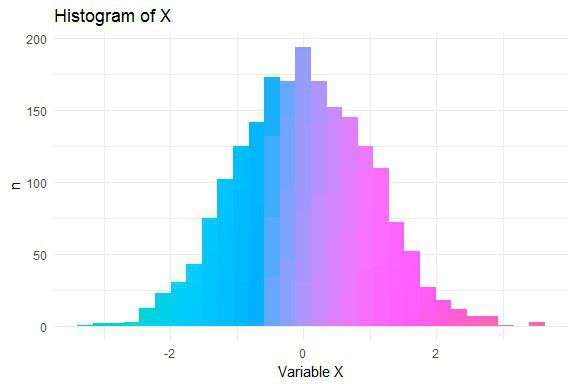## The data

Let’s simulate data for a continuous variable `x` in a data frame `d`:

```set.seed(070510)
d <- data.frame(x = rnorm(2000))

#>            x
#> 1  1.3681661
#> 2 -0.0452337
#> 3  0.0290572
#> 4 -0.8717429
#> 5  0.9565475
#> 6 -0.5521690
```

## Basic Histogram

Create the basic ggplot2 histogram via:

```library(ggplot2)

ggplot(d, aes(x)) +
geom_histogram()
```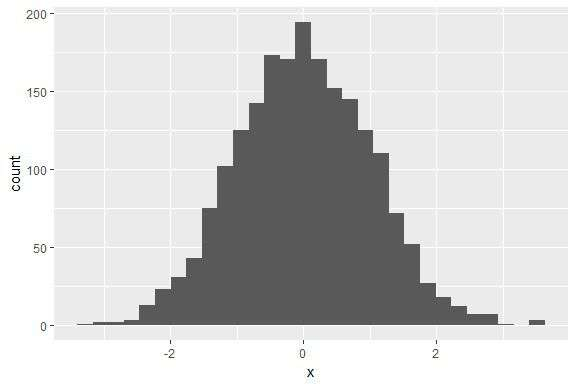Time to jazz it up with colour! The method I’ll present was motivated by my answer to this StackOverflow question.

We can add colour by exploiting the way that ggplot2 stacks colour for different groups. Specifically, we fill the bars with the same variable (`x`) but `cut` into multiple categories:

```ggplot(d, aes(x, fill = cut(x, 100))) +
geom_histogram()
```What the…

Oh, ggplot2 has added a legend for each of the 100 groups created by cut! Get rid of this with `show.legend = FALSE`:

```ggplot(d, aes(x, fill = cut(x, 100))) +
geom_histogram(show.legend = FALSE)
```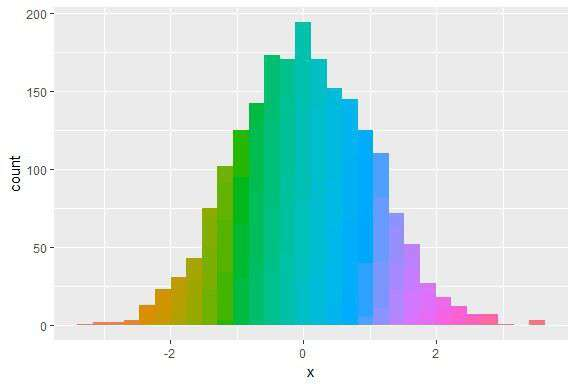Not a bad starting point, but say we want to tweak the colours.

For a continuous colour gradient, a simple solution is to include `scale_fill_discrete` and play with the range of hues. To get your colours right, get familiar with the hue scale.

For example, here we’ll tweak the colours to range from blue to red:

```ggplot(d, aes(x, fill = cut(x, 100))) +
geom_histogram(show.legend = FALSE) +
scale_fill_discrete(h = c(240, 10))
```Seems a little dark. Tweak chroma and luminance with `c` and `l`:

```ggplot(d, aes(x, fill = cut(x, 100))) +
geom_histogram(show.legend = FALSE) +
scale_fill_discrete(h = c(240, 10), c = 120, l = 70)
```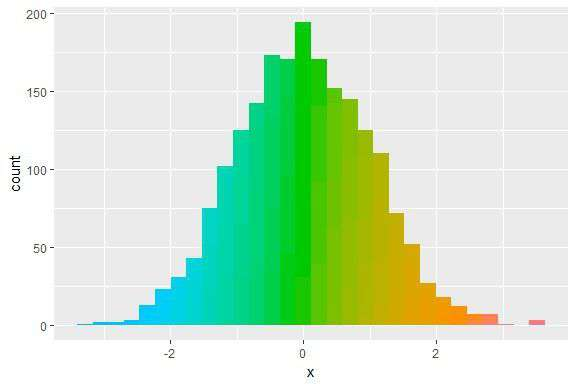## Final touches

The final touches are to set the theme, add labels, and a title:

```ggplot(d, aes(x, fill = cut(x, 100))) +
geom_histogram(show.legend = FALSE) +
scale_fill_discrete(h = c(240, 10), c = 120, l = 70) +
theme_minimal() +
labs(x = "Variable X", y = "n") +
ggtitle("Histogram of X")
```Now have fun tweaking the colours!

```p <- ggplot(d, aes(x, fill = cut(x, 100))) +
geom_histogram(show.legend = FALSE) +
theme_minimal() +
labs(x = "Variable X", y = "n") +
ggtitle("Histogram of X")

p + scale_fill_discrete(h = c(180, 360), c = 150, l = 80)
```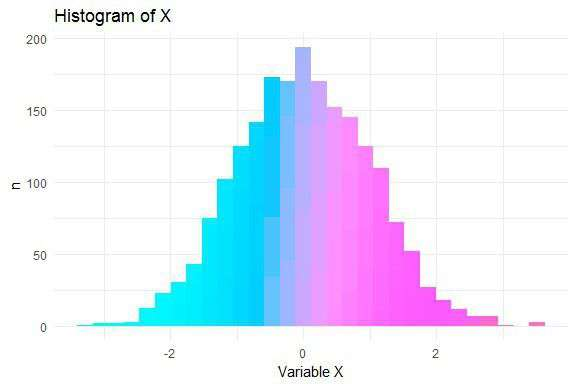```p + scale_fill_discrete(h = c(90, 210), c = 30, l = 50)
```## Sign off

Thanks for reading and I hope this was useful for you.

For updates of recent blog posts, follow @drsimonj on Twitter, or email me at [email protected] to get in touch.

If you’d like the code that produced this blog, check out the blogR GitHub repository.